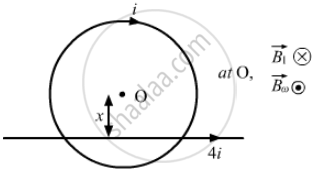Department of Pre-University Education, KarnatakaPUC Karnataka Science Class 12
Advertisement Remove all ads

# A Circular Loop of Radius R Carries a Current I. How Should a Long, Straight Wire Carrying a Current 4i Be Placed in the Plane of the Circle So that the Magnetic Field at the Centre Becomes Zero? - Physics

Short Note

A circular loop of radius r carries a current i. How should a long, straight wire carrying a current 4i be placed in the plane of the circle so that the magnetic field at the centre becomes zero?

Advertisement Remove all ads

#### Solution

Given:
Magnitude of current = i
Radius of the loop = r
Magnetic field due to the loop at its centre,

$B {}_l = \frac{\mu_0 i}{2r}$
Let a straight wire carrying 4 i current be placed at a distance x from the centre such that the magnetic fields of the loop and the wire are of equal magnitude but in opposite direction at O.Magnetic field due to the wire at the centre of the loop,

$B_w = \frac{\mu_0 4i}{2\pi x}$
According to the question,

$B_l = B_w$
$\Rightarrow \frac{\mu_0 i}{2r} = \frac{\mu_0 4i}{2\pi x}$
$\Rightarrow x = \frac{8r}{2\pi} = \frac{4r}{\pi}$

This means that the wire is placed $\frac{4r}{\pi}$  from the centre of the loop (as shown in the figure).

Concept: Magnetic Field on the Axis of a Circular Current Loop
Is there an error in this question or solution?
Advertisement Remove all ads

#### APPEARS IN

HC Verma Class 11, Class 12 Concepts of Physics Vol. 2
Chapter 13 Magnetic Field due to a Current
Q 43 | Page 252
Advertisement Remove all ads
Advertisement Remove all ads
Share
Notifications

View all notifications

Forgot password?